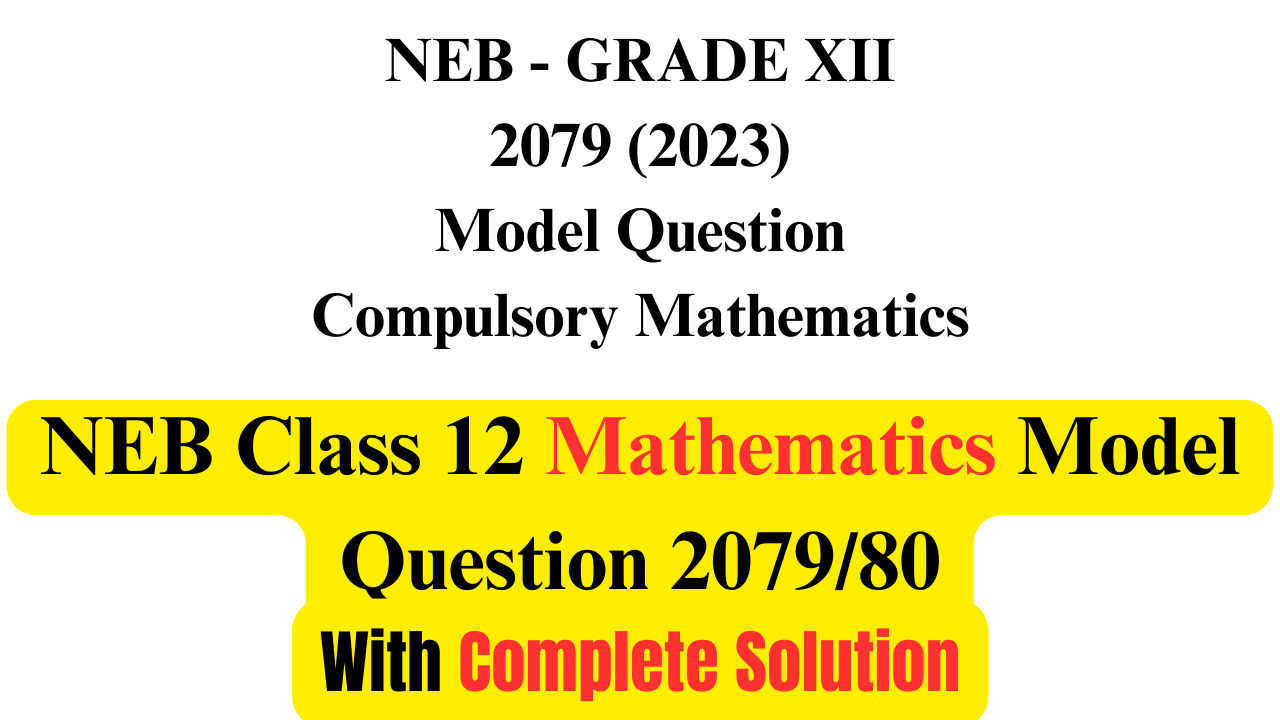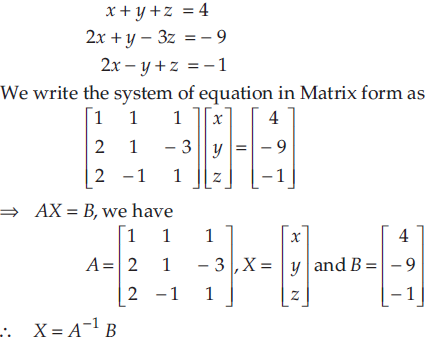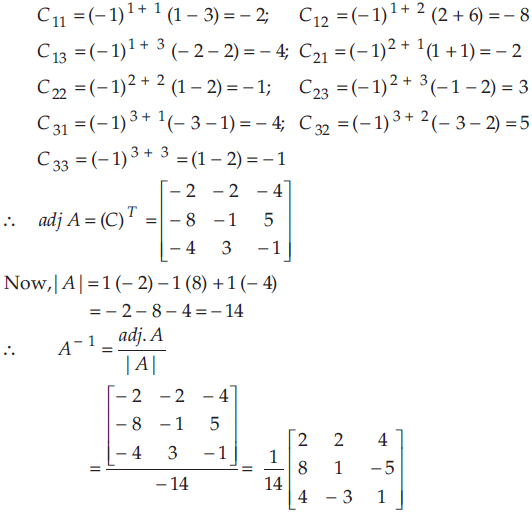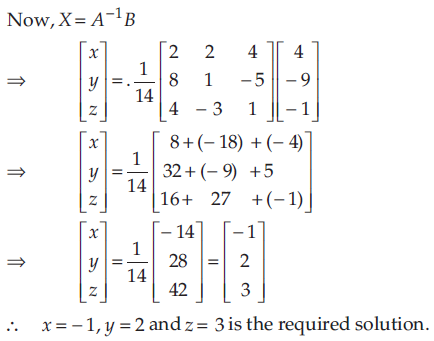# NEB Class 12 Math Model Question 2080 With Solution

Class 12 Mathematics Model Questions 2079/2080 Solution. Latest NEB Model Question & Complete Answer Solution of Math for Class 12 Board Exam 2080.NEB Class 12 Math Model Question Paper 2079 With Solution

In This Post, We Provide Complete Class 12 Mathematics  Model Question and Answer Solution 2080 issued by NEB.

## Class 12 Model Question 2079

Class 12 Mathematics Model Questions 2079/80 With Solution. Latest NEB Model Question & Complete Answer Solution of Math for Class 12 Board Exam 2080. NEB Class 12 Model Question 2079/80 is given as:

## Class 12 Model Question Solution 2079

NEB Class 12 Model Question Solution 2079/80 is given as:

Disclaimer:
Answers mentioned here are not solved by teachers. These are the solutions written by a student of Grade 12. If you found some of the solutions mistake please let me know in the comment section below. I took help of book, search engines, and teachers to solve the set. However, the language or process of solving the questions might be informal and in examinations, you might have to add little more language and show more calculations than what has been done here. So, I highly encourage you to view these solutions as guide rather than just copying everything mentioned here.

Candidates are required to give their answers in their own words as far as practicable. The figures in the margin indicate full marks.

Time: 3hrs                                                                  Full Mark:75

Attempt all questions.

### Group "A"

1) What is an arrangement of the n natural numbers called?

• A) Induction
• B) Permutation
• C) Combination
• D) Expectation

Ans: B) Permutation

2) Let 1,w,w² be the cube roots of unity. Under which operation is the set A={1,w,w²} closed?

• B) Subtraction
• C) Multiplication
• D) Division

Ans: C) Multiplication

3) What is the domain of sin^-1x?

• A) x≥1 or x≤-1
• B) (,infinity)
• C) -1<x<1
• D) -1≤x≤1

Ans: D) -1≤x≤1

4) ABCD is a parallelogram. Which one of the following represents area of the parallelogram?

• A) ﻿﻿﻿Magnitude of vector product of two vectors along AB and BD.
• ﻿﻿﻿B) Magnitude of vector product of two vectors along AB and DC.
• C) ﻿﻿﻿Magnitude of vector product of two vectors along AC and BC.
• D) ﻿﻿﻿Magnitude of vector product of two vectors along AB and AD.

Ans: A) ﻿﻿﻿Magnitude of vector product of two vectors along AB and BD.

5) If a conic section has eccentricity(e) = (√a^2-b^2)/a, what is the equation of that conic section?

• A)(x^2/a^2)-(y^2/b^2)=1
• B) (x^2/a^2)+(y^2/b^2)=1
• C) (x^2/a^2)+(y^2/a^2)=1
• D)(x^2/b^2)+(y^2/b^2)=1

Ans: B) (x^2/a^2)+(y^2/b^2)=1

6) If cosӨ =−1/for integer (n), what is the general value of Ө ?

• A) 2nπ ±2π/3
• B) nπ + (−1)^π/3
• C) nπ −π/3
• D) nπ +π/3

Ans: A) 2nπ ±2π/3

7) Let A and B be two dependent events. If P (A) =1/2, P (B) = 3/4 and P(AnB) =2/5, what is the value of P(A/B)?

• A) equal to P(B/A)
• B) equal to P(A)
• C) less than P(A A B)
• D) less than P(B/A)

Ans:D) Less than P(B/A)

8) The edge of a cube increases from 10 cm to 10.025 cm. What would be the approximate increment in volume?

• A) 103 cm^3?
• B) 10.0253 cm^3?
• C) 7.5187 cm^3?
• D) 7.5 cm^3?

Ans: D) 7.5 cm^3?

9) What is the integrating factor of the differential equation cos'× dy + y =1°

• A) tan x
• B) e^tanx
• C) sec^2 x
• D)e^sec1x

Ans: B) e^tanx

10) What is the number of solutions of the system of linear equations × + y = 5 and x + y = 7?

• A) One solution
• B) No solution
• C) Infinite solutions
• D) More than one solution

Ans: B) No solution

11) Forces P and Q are acting along ceiling and floor of a rectangular room. What is the nature of the forces?

• A) Like
• B) unlike
• C) collinear
• D) parallel

Ans: B) unlike

### Group "B"

12) For any positive integer n, (a+x)n = C0an + C1an-1x + C2an-2x2+……+cnxn.

a) How many terms are there in the expression?

b) Write binomial coefficient in the expansions?

c) Write the general term of the expansion.

d) Write the relations among C (n, r-1), C (n+1, r) and C (n, r).

e) What is the value of C0+C1+C2 +…+Cn?

Solution:

(a) There will be (n+1) numbers of terms in the expansion of (a+x)n = C0an + C1an-1x + C2an-2x2+……+cnxn.

(b) The binomial coefficient in the expansion of (a+x)n = C0an + C1an-1x + C2an-2x2+……+cnxn are: C0, C1an-1, C2an-2,……Cn

(c) The general terms of the expansion is nCran-rxr.

(d) The relations among C (n, r-1), C (n+1, r) and C (n, r) is nr + nCr-1 = n+1Cr.

(e) The value of C0+C1+C2 +…+Cn is 2n.

13) a) Using the principle of mathematical induction, show that: 1+2+3+…+n < $\frac{1}{8}$(2n+1)2.

b) Find the quadratic equation whose one of the cube roots is 2+$\sqrt{3}$

Solution:

Step 1: Let n = 1

P(1): 1<$\frac{1}{8}$[2(1)+1]2 is true.

Hence, the statement is true for n=1

Step 2: Assume that the statement is true for n=k

P (k) : 1 + 2 + 3 + … + k < $\frac{1}{8}$ (2k+1)2

Step 3: Prove that the statement is true for n=k+1

We need to prove that:

1 + 2 + 3 + …. + (k+1) < $\frac{1}{8}$ [(2k+1)2] + k+1

< $\frac{1}{8}$ [4k2+4k+1] + 8 ×$\frac{1}{8}$ × (k+1)

<$\frac{1}{8}$ [4k2+4k+1+8k+8]

<$\frac{1}{8}$ [4k2+12k+9]

<$\frac{1}{8}$ [2k+3]2

<$\frac{1}{8}$[2(k+1)+1]2

(b) For the quadratic equation if one root (α)= 2 +$\sqrt{3}$

Another Root (β) = 2 - $\sqrt{3}$

Sum of Roots (α+ β) = 2 - $\sqrt{3}$ + 2 +$\sqrt{3}$ =4

Products of Roots (α × β) = (2 - $\sqrt{3}$)(2 + $\sqrt{3}$) = 1

∴Quadratic equation : x2 -(α+ β)x + (α × β) =0

∴ x2−4x+1=0 is required equations.

14) a) Given y = sin-1x and y>0, express cos y and tan y in terms of x.

b) If $\stackrel{\to }{a}$, $\stackrel{\to }{b}$, and $\stackrel{\to }{c}$ are any three vectors such that $\stackrel{\to }{a}$ × $\stackrel{\to }{b}$ = $\stackrel{\to }{a}$ × $\stackrel{\to }{c}$ for $\stackrel{\to }{a}$ ≠(0,0). Show that: $\stackrel{\to }{b}$ = $\stackrel{\to }{c}$.

Solution:

(a) Given:

y = sin-1x

or, x = sin y

We have, Cos2y + Sin2y = 1

Or, Cos2y=1- Sin2y

Or, Cos2y = 1-x2

Thus, Cos y = $\sqrt{1-{x}^{2}}$

And, $\mathrm{Tan}y=\frac{\mathrm{sin}x}{\mathrm{cos}x}=\frac{x}{\sqrt{1-{x}^{2}}}$

(b) Given:

$\stackrel{\to }{a}$ × $\stackrel{\to }{b}$ = $\stackrel{\to }{a}$ × $\stackrel{\to }{c}$

If Cross product is equal then their magnitude is also equal.

|$\stackrel{\to }{a}$ × $\stackrel{\to }{b}$ | = | $\stackrel{\to }{a}$ × $\stackrel{\to }{c}$|

Or, |$\stackrel{\to }{a}$|  |$\stackrel{\to }{b}$| Sinθ = | $\stackrel{\to }{a}$| |$\stackrel{\to }{c}$| Sinθ

Or, |$\stackrel{\to }{b}$ |=|$\stackrel{\to }{c}$ |

$\stackrel{\to }{b}$ = $\stackrel{\to }{c}$

15) The price in Rupees (X) and demand in unit (Y) of 6 days of a week is given as:

 X 10 12 13 12 16 15 Y 40 38 43 45 37 43

Calculate the Pearson’s Coefficient of Correlation and the regression coefficients of X on Y.

Solution:

 x y xy x2 y2 10 40 400 100 1600 12 38 456 144 1444 13 43 559 169 1849 12 45 540 144 2025 16 37 592 256 1369 15 43 645 225 1849 Σx=78 Σy=246 Σxy=3192 Σx2=1038 Σy2=10136

Value of n = 6

r=nxyxynx2(x)2.ny2(y)2

r=6×319278×2466×1038(78)2.6×10136(246)2

r=0.36144.300

r=1.7132

Now, Regression Coefficient of x on y is

bxy=nxyxy(y)2ny2

bxy=6×319278×24624626×10136

bxy=36300

bxy=0.12

16) a) Define Hospital’s rule.

b) Write the slope of the tangent and normal to the curve y =f(x) at (x1,y1).

c) Write the integral of $\int \frac{1}{{x}^{2}+{a}^{2}}dx$.

d)What is the integral of $\int \mathrm{sinh}x.dx$

Solution:

(a) If f(x) and g(s) are two function then their derivatives f’(x) and g’(x) are continuous at x=0 and if f(a) =g(a), then,

$\underset{x\to a}{lim}\frac{f\left(x\right)}{g\left(x\right)}=\underset{x\to a}{lim}\frac{{f}^{\prime }\left(x\right)}{{g}^{\prime }\left(x\right)}=\frac{{f}^{\prime }\left(a\right)}{{g}^{\prime }\left(a\right)}$ When g’(a)≠0.

(b) The slope of tangant to the curve y=f(x) at (x1,y1) is

dydx=f(x)

dydx(x1,y1)=f(x1)

Then, Slope of normal to y=f(x) at ((x1,y1)) is

F’(x). m = -1

Thus, m=$-\frac{dx}{dy}$

(c) $\int \frac{1}{{x}^{2}+{a}^{2}}dx$=$\frac{1}{a}{\mathrm{tan}}^{-1}\frac{x}{a}+C$

(d) $\int \mathrm{sinh}x.dx$ = coshx +C

17) a) Solve: $\frac{dy}{dx}=\frac{y}{x}$

b) Verify Rolle’s Theorem for f(x) = x2+3x-4 in [-4,1].

Solution:

dydx=yx

dyy=dxx

Integrating both sides:

ln(y) = ln(x) +C

or, ln(y) = ln(x) + ln(C)

Thus, y=xc

(b) f(x) = x2+3x-4 in [-4,1]

(i) Here f(x) is a polynomial function so it is continuous at [-4,1].

(ii) f’(x) = 2x+3

f(x) is differentiable in (-4, 1).

(iii) f(-4) = 0, f(1) = 0

Thus, f(a) = f(b).

All the condition of Rolle’s Theorem are verified. So there exists at least a point C ε (-4, 1).

Such that: f’(C) =0.

18) Using simplex method, maximize P (x, y) = 15x+10y subject to 2x+y≤10, x+3y≤10, x, y≥0.

Solution:

Maximize P (x, y) = 15x+10y

Subject to:

2x+y≤10

x+3y≤10

x, y≥0

Let S1 and S2 be two non-negative slack variables. Then, the standard form of the above LPP is:

2x+y+r =10

x+3y+s=10

-15x-10y+P=0

The initial simplex tableau for above LLP is

 Basic Variable x y r s p Constant r 2 1 1 0 0 10 s 1 3 0 1 0 10 -15 -10 0 0 1 0

In the initial Simplex tableau the most negative entry is -15 so the x-column is pivot column. Also, $\frac{10}{2}$=5 is the smallest positive value. Thus, r-row with element 2 on x column is the pivot element.

Dividing First Row by 2

 Basic Variable x y r s p Constant r 1 ½ ½ 0 0 5 s 1 3 0 1 0 10 -15 -10 0 0 1 0

R2à R2-R1 and R3à R3+15R1

 Basic Variable x y r s p Constant r 1 ½ ½ 0 0 5 s 0 $\frac{5}{2}$ -½ 1 0 5 0 -$\frac{5}{2}$ $\frac{}{}$152 0 1 75

Here last row still has -ve entry. So y is pivot column and s-row is the pivot row with $\frac{5}{2}$ as pvot element.

R2à $\frac{2}{5}$R2

 Basic Variable x y r s p Constant r 1 ½ ½ 0 0 5 s 0 1 -$\frac{1}{5}$ $\frac{}{}$25 0 2 0 -$\frac{5}{2}$ $\frac{}{}$152 0 1 75

Again, R3 à R3+$\frac{5}{2}$R2 and R1àR1- ½ R2

 Basic Variable x y r s p Constant r 1 ½ $\frac{3}{5}$ -$\frac{1}{5}$ 0 4 s 0 1 -$\frac{1}{5}$ $\frac{}{}$25 0 2 0 0 7 1 1 80

Since there is no negative entry in last row. Thus Maximum Solution is obtained.

Pmax=80 at x=4 and y=2.

19) A particle is projected with a velocity ‘v’ and greatest height is ‘H’, prove the horizontal range R is: R = 4$\sqrt{H\left(\frac{{v}^{2}}{2g}-H\right)}$.

Solution:

Let β be angle of projection. Then,

H=v2sin2β2g

R=v2sin2βg

Now,

v22gH

=$\frac{{v}^{2}}{2g}-\frac{{v}^{2}{\mathrm{sin}}^{2}\beta }{2g}$

=$\frac{{v}^{2}}{2g}\left(1-{\mathrm{sin}}^{2}\beta \right)$

=$\frac{{v}^{2}}{2g}{\mathrm{cos}}^{2}\beta$

And,

R.H.S of Question:

4$\sqrt{H\left(\frac{{v}^{2}}{2g}-H\right)}$ = $4.\frac{v\mathrm{sin}\beta .\mathrm{cos}\beta }{2g}$

4v2sin2β2g.v22gcos2β

4.v2sinβ.cosβ2g

$\frac{}{}$v2.2sinβ.cosβg

$\frac{}{}$v2sin2βg

=R

=L.H.S

OR

The cost function C(x) in thousands of rupees for producing x units of math’s textbooks is given by C(x)=30+20x-0.5x2 , 0≤x≤15.

a) Find the marginal cost of Function.

b) Find the marginal cost for producing 12000 math’s textbooks.

Solution:

C(x)=30+20x-0.5x2, x, y≥0

(a) Marginal Cost of Function = C‘(x)=0+20-x

(b) Marginal cost = 20-x=20-12=Rs. 8 (in thousands)

## Group "C"

20) a) Using matrix methods, solve the following system of linear equations: x+y+z = 4, 2x+y-3z = -9,2x-y+z = -1

b) Apply De-Moiver’s theorem to find the value of [2(𝑐𝑜𝑠15° + 𝑖𝑠𝑖𝑛15°)] 6

c) Prove that: $\left(1+\frac{1}{1!}+\frac{1}{2!}+\frac{1}{3!}+...\right)\left(1-\frac{1}{1!}+\frac{1}{2!}-\frac{1}{3!}+...\right)=1$

Solution:

(a)(b) De Moiver’s Theorem states that, {r(cosθ+isinθ)}n=rn(cosnθ+isinnθ).

∴[2(𝑐𝑜𝑠15° + 𝑖𝑠𝑖𝑛15°)] 6 = 26 [cos90 + i sin90] = 64i

(c) We Know  ex$1+\frac{x}{1!}+\frac{{x}^{2}}{2!}+\frac{{x}^{3}}{3!}+...$

Put x = 1

e1=$1+\frac{1}{1!}+\frac{1}{2!}+\frac{1}{3!}+...$(i)

Put x = -1

e-1=$1-\frac{1}{1!}+\frac{1}{2!}-\frac{1}{3!}+...$(ii)

Taking LHS of Question:

(1+11!+12!+13!+...)(111!+12!13!+...)

=e × e-

=1

=R.H.S

21) a) Find the direction cosines of the line joining the points (4,4,-10) and (-2,2,4).

b) Find the angle between the two diagonals of a cube.

c) Find the vertices of the conic section: 16(y-1)2 -9(x-5)2 = 144.

Solution:

(a) Given Points: P(4,4,-10) and Q(-2,2,4)

Direction ratios of PQ = -2-4, 2-4, 4-(-10)=-6, -2, 14

PQ=$\sqrt{{\left(-6\right)}^{2}+{\left(-2\right)}^{2}+{\left(14\right)}^{2}}=2\sqrt{59}$

Thus, Direction cosines are: $\frac{-6}{2\sqrt{59}},\frac{-2}{2\sqrt{59}},\frac{14}{2\sqrt{59}}$

(b) Let OABCDEFG be a cube with vertices as below

O(0,0,0),A(a,0,0),B(a,a,0),C(0,a,0),D(0,a,a),E(0,0,a),F(a,0,a),G(a,a,a)

There are four diagonals OG,CF,AD  and BE for the cube.

Let us consider any two say OG and AD

We know that if A(x1​,y1​,z1​) and B(x2​,y2​,z2​) are two points in space then

AB=(x2​−x1​)i+(y2​−y1​)j+(z2​−z1​)k

⇒OG=(a−0)i+(a−0)j+(a−0)k=ai+aj+ak

Therefore ∣$\stackrel{\to }{OG}$∣=$\sqrt{{a}^{2}+{a}^{2}+{a}^{2}}=a\sqrt{3}$

And,

$\stackrel{\to }{AD}$ = $\sqrt{{\left(-a\right)}^{2}+{a}^{2}+{a}^{2}}=a\sqrt{3}$

OG.AD=a2+a2+a2=a2

We know that angle between two vectors $\stackrel{\to }{OG},\stackrel{\to }{AD}$ is given by θ = ${\mathrm{cos}}^{-1}\frac{\stackrel{\to }{OG}.\stackrel{\to }{AD}}{|\stackrel{\to }{OG}|.|\stackrel{\to }{AD}|}$

=${\mathrm{cos}}^{-1}\frac{{a}^{2}}{a\sqrt{3}.a\sqrt{3}}$

=${\mathrm{cos}}^{-1}\frac{1}{3}$

Thus, angle between two vectors $\stackrel{\to }{OG},\stackrel{\to }{AD}$ is ${\mathrm{cos}}^{-1}\frac{1}{3}$

(c) Given:

16(y-1)2 -9(x-5)2 = 144….(i)

Dividing Both Sides by 144.

(y1)29(x5)216=1

Comparing with $\frac{{\left(y-h\right)}^{2}}{{a}^{2}}-\frac{{\left(x-k\right)}^{2}}{{b}^{2}}=1$

We Get:

h=1, k=5, a=3, b=4

Thus, Vertex = (h±a, k) = (1±3, 4) =(4,4) 0r (-2, 4)

22) a) If the limiting value of $\frac{f\left(x\right)-5}{x-3}$ at x= 3 is 2 by using L’ Hospital’ rule, find the appropriate value of f(x).

b) Write any one homogeneous differential equation in (x,y) and solve it.

c) The concept of anti-derivative is necessary for solving a differential equation. Justify this statement with example.

Solution:

(a)$\begin{array}{l}\frac{\underset{x\to 3}{lim}f\left(x\right)-5}{x-3}=2\\ or,\underset{x\to 3}{lim}f\left(x\right)-5=2x-6\\ or,\underset{x\to 3}{lim}f\left(x\right)=2x-1\end{array}$

(b)  Homogenous differential Equation in (x,y) is $\frac{dy}{dx}=\frac{y}{x}+1$

Solving This Equation:

dydx=yx+1

Put y =vx, then $\frac{dy}{dx}=v+x\frac{dv}{dx}$

$\begin{array}{}\end{array}$yx+1=v+xdvdxor,v+1=v+xdvdxor,1=xdvdxor,dxx=dv

Integrate both sides

v = log x + log c

or, v = log cx

Replacing v

yx=logcx

∴ y = x log cx

(c) The concept of anti-derivatives is necessary for solving differential equation. Let’s understand this statement through the following example:

Consider a differential Equation $\frac{dy}{dx}=\frac{x}{y}$

i.e., y.dy = x.dx

Let’s integrate this on both sides:

y.dy=x.dx

y22=x22+C

Or, y2=x2+2C

i.e., y2=x2+C

Math Model Question Solution Youtube Video

The below answer provided for question 15 is incorrect. you can check correct answer from above but

Formula n€y²-(€y)2 hunxa formula

ans -0.12 aau xa.

Group "C"

Getting Info...

Iswori Rimal is the author of iswori.com.np, a popular education platform in Nepal. Iswori helps students in their SEE, Class 11 and Class 12 studies with Complete Notes, important questions and other study materials.

1. Can you please, check question number 15 (last part ) I think that one is incorrect.
1. yes yes; wrong xa jasto xa; thankyou;
2. aaba hernuhos ta answer melya xa ke nai
2. Mcq no.4 ko answer d hunxa hola
1. The correct answer is A) ﻿﻿﻿Magnitude of vector product of two vectors along AB and BD.

To see why, let's recall that the area of a parallelogram can be calculated as the magnitude of the cross product of two adjacent sides. In this case, we can choose AB and AD as the adjacent sides, and calculate the cross product of the vectors along these sides:

However, we can also express AD as the sum of AB and BD (since AB and AD are adjacent sides of the parallelogram), giving us:

Substituting this into the expression for the cross product, we get:

Area = |AB x (AB + BD)|

Expanding the cross product using the distributive property, we get:

Area = |AB x AB + AB x BD|

Since the cross product of a vector with itself is zero (i.e., v x v = 0 for any vector v), we can simplify this to:

Area = |AB x BD|

Therefore, the area of the parallelogram can be calculated as the magnitude of the vector product of two vectors along AB and BD, which is option A.
3. How can I contact you? Mam/sir
1. contact with mail: [email protected] or fb facebook
4. tyo 13 ko b ma another root 2-√3 kina lekheko? question le kei clarify nei gareko xaina arko root yesto hunxa vanera, eetikai ans milauna lai testo lekhna painxa ra?
1. if one root is 2 +√3 then another will be 2-√3 ; We can then use the fact that complex roots of a quadratic equation come in conjugate pairs to find the other root